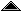# First Order Predicate Logic

 This is a semi-formal specification of a simplified version of the predicate logic called Q by Hunter in [Hunter71]. I call the specification semi-formal because I am not supplying a definition of the metalanguage in which it is written. The system is a classical predicate logic with two propositional connectives (not and implies) presented as a "Hilbert style" axiom system in which there are two inference rules (modus ponens and generalisation), and four axiom schemata.

##::= Var (variable) ::= | , ::= P() (a predicate applied to a list of terms) ::= (a predicate applied to terms) |(negation) |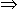(implication) |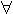. (universal quantification)

##An interpretation consists of a set of elements, which is called the domain of the interpretation, together with an assignment to each predicate letter of a set of finite sequences of elements from the domain. A valuation is an assignment to each variable of an element in the domain of interpretation.

The truth value of a formula f under an interpretation i and valuation v is fpeval i v f, where fpeval is a (higher order) function taking three successive arguments, and is defined as follows (function application is shown as juxtaposition, brackets are not necessary for simple arguments):

 fpeval i v (P x seqt)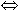(tseval v seqt)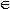(i x) fpeval i v (f)(fpeval i v f) fpeval i v (f1f2)(fpeval i v f1)(fpeval i v f2) fpeval i v (x. f)ydom(i). fpeval i (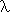z. if z=x then y else v z) f
Where tseval evaluates a sequence of terms using a valuation v yielding a sequence of elements in the domain of interpretation, and may be defined:
 tseval v nil = nil tseval v ((Var x), seqt) = ((v x),(tseval v seqt))

 A formula is valid (and hence true) if it is true under every interpretation/valuation combination and contradictory (and hence false) if it is false under every interpretation/valuation.

##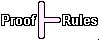There are just two inference rules, modus ponens and generalisation, and six axiom schemata, as follows.

Modus Ponens
 From two theorems, one of the form:A and the other of the form:AB where A and B are arbitrary formulae, the conclusion:B may be derived.

Generalisation
 From a theorem of the form:A infer:v. A for any variable v.

Axiom Schemata
All instances of the following schemata are theorems:A(BA)((A(BC))((AB)(AC)))((AB)(BA))(x. A)A [t/v] (renaming bound variables as necessary)(Ax. A) (unless x occurs free in A)(x. AB)((x. A)(x. B))©created 1997/10/18 modified 1997/10/25